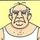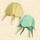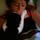## General Question#### How do I do this statistical calculation?

Asked by srmorgan (6768) September 22nd, 2013
2 responses
“Great Question” (0)

I took statistics over 35 years ago and I don’t remember this.

I have a population of data, 130 instances. My average is 1500 and my standard deviation =stdevp () is 611.
Which values are one standard deviation away from the mean and which values are two standard deviations away from the mean.

I can’t find a 2nd standard deviation function in Excel.

ThanksFollow this questionSend to a friend!Topic:
Observing members: 0Composing members: 02 standard deviations for this population is 611×2 = 1222

drhat77 (6197)“Great Answer” (2)The good dr up there is correct. So the values that are 1 standard deviation away from the mean are the numbers between (mean – 1 standard deviation) and (mean + 1 standard deviation, and the values that are 2 standard deviations away are between (mean – standard deviation*2) and (mean + standard deviation*2).

Mean +/- 1 SD = 889 to 2111
Mean +/- 2 SDs = 278 to 2722

nikipedia (28071)“Great Answer” (3)